### Taichi Cookbook 001

Hey guys! Welcome to my first Taichi cooking session!

From time to time, I hear our users ask questions like "how can I make my code cleaner and more straightforward" or "how can I further optimize the performance of my Taichi programs". So, I decided to share some very practical tips I myself often use when coding with Taichi, as well as a new feature `ti.dataclass`. Hopefully, you can make good use of them the next time you `ti.init`.

Before you proceed, make sure you are using the latest version of Taihci (v1.0.4). Upgrade and view our classy demos:

``pip install --upgrade taichiti gallery``

If you have not installed Taichi, you can also run the demos below in Colab.

## Five tips

### Tip 1: Auto-debug out-of-bound array accesses

The array access violation issue is quite common during low-code programming (such as C++ and CUDA), and more often than not, a program would proceed regardless. You would not even realize it until you ended up with a wrong result. Even if, with a stroke of luck, you saw a segmentation fault triggered, you would find it hard to debug. Taichi solves this problem by providing an auto-debugging mode: just set `debug=True` when initiating Taichi. For example:

``import taichi as titi.init(arch=ti.cpu, debug=True)f = ti.field(dtype=ti.i32, shape=(32, 32))@ti.kerneldef test() -> ti.i32:    return f[0, 73]    print(test())``

And you will see an error appear: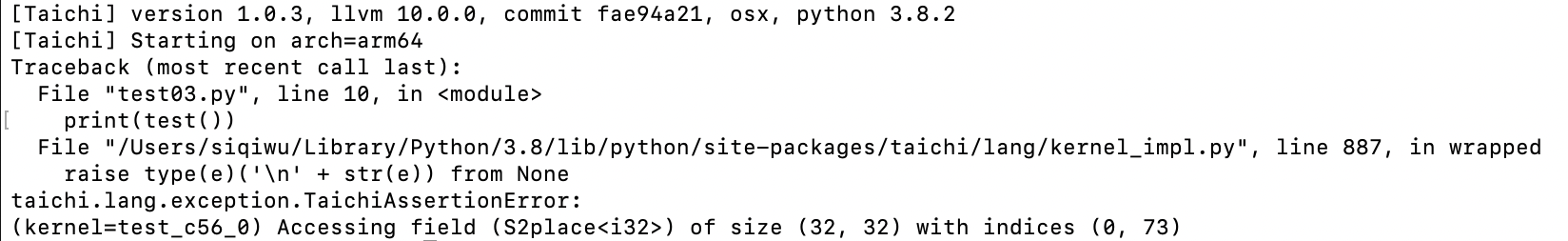To sum up:

1. Bound checks are not available until you enable `debug=True`.

2. Only `ti.cpu` and `ti.cuda` are supported (you should switch to CPU/CUDA for bounds checking if you are using other backends).

3. Program performance may worsen after `debug=True` is turned on.

### Tip 2: Access a high-dimensional field by indexing integer vectors

It can be cumbersome to use `val[i, j, k, l]` to access an element in a high-dimensional field. Is there an easier way to do that? Well, we can index an integer vector directly (and conduct math operations based on such vectors) like this:

``import taichi as tiimport matplotlib.pyplot as pltimport mathti.init(arch=ti.cpu)n = 512img = ti.field(dtype=ti.i32, shape=(n, n))img_magnified = ti.field(dtype=ti.i32, shape=(n, n))@ti.kerneldef paint():    for I in ti.grouped(img):        f = (I / n) * math.pi * 10        img[I] = ti.sin(f) + ti.cos(f)        paint()plt.imshow(img.to_numpy())plt.show()@ti.kerneldef magnify():    for I in ti.grouped(img_magnified):        img_magnified[I] = img[I // 2]        # equivalent to    # for i, j in img_magnified:    #     img_magnified[i, j] = img[i // 2, j // 2]magnify()    plt.imshow(img_magnified.to_numpy())plt.show()``

And run the program: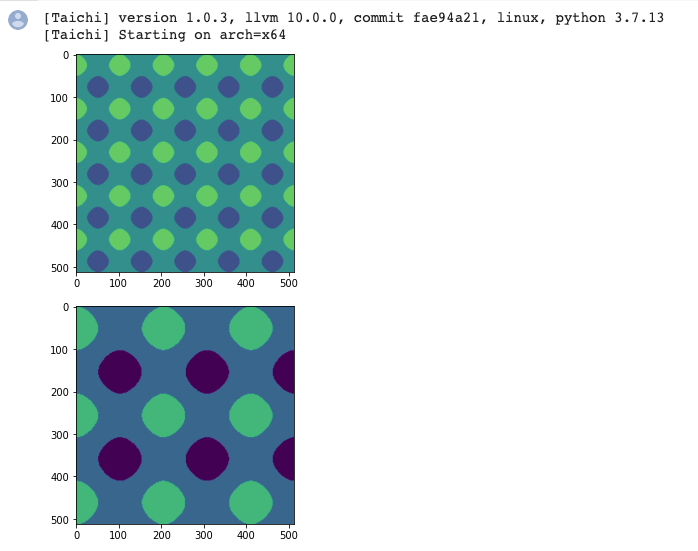To sum up:

1. `for I in ti.grouped(img)`: Make sure you use `ti.grouped` to pack the index into `ti.Vector`.

2. If it is a floating-point vector, make sure you use `I.cast(ti.i32)` to cast it to an integer; otherwise, a warning would occur.

3. The point of this tip is that your code becomes dimension-independent. You can apply the same set of code for either 2D or 3D.

### Tip 3: Serialize the outermost for loop

By default, Taichi automatically parallelizes the for loop at the outermost scope, but sometimes some programs need to be serialized. In this case, you just need `ti.loop_config(serialize=True)`:

``import taichi as titi.init(arch=ti.cpu)n = 1024val = ti.field(dtype=ti.i32, shape=n)val.fill(1)@ti.kerneldef prefix_sum():    ti.loop_config(serialize=True)    for i in range(1, n):        val[i] += val[i - 1]prefix_sum()print(val)``

And you will get the right result:To sum up:

1. `ti.loop_config(serialize=True)` decorates the outermost for loop that immediately follows it.

2. `ti.loop_config` works only for the range-for loop at the outermost scope.

3. Inner for loops are serialized by default.

In addition, you can try warp-level intrinsics to accelerate prefix sum if you are using CUDA: https://github.com/taichi-dev/taichi/issues/4631

### Tip 4: Interact with Python libraries, such as NumPy

"I really want to convert the output to the data types supported by NumPy so I can paint with Matplotlib or develop deep learning models with PyTorch!"

Taichi provides a solution:

``import taichi as tiimport matplotlib.pyplot as pltti.init(arch=ti.cpu)n = 8192factors = ti.field(dtype=ti.i32, shape=n)@ti.kerneldef number_of_factors():    for i in range(1, n):        counter = 0        for j in range(1, int(ti.floor(ti.sqrt(i))) + 1):            if i % j == 0:                if j * j == i:                    counter += 1                else:                    counter += 2        factors[i] = counternumber_of_factors()plt.plot(factors.to_numpy(), '.')# for i in range(n):#     print(i, factors[i])plt.show()factors.to_torch()``

I tried it out with Matplotlib and it went well: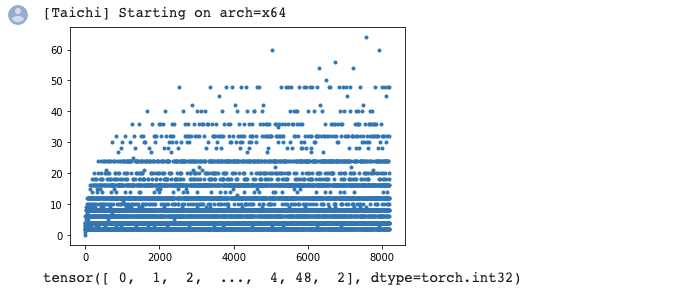### Tip 5: Analyze performance with Taichi Profiler

"It takes a long time to run my program, but how can I figure out which Taichi kernel is the most time-consuming?"

Well begun is half done. It is crucial to locate the bottleneck before you start optimization, and Taichi's Profiler can do that for you:

``import taichi as titi.init(arch=ti.cpu, kernel_profiler=True)f = ti.field(ti.i32, shape=(32, 32))@ti.kerneldef foo():    for i in range(400000):        f[0, 0] += i            for i in range(100000):        f[0, 1] += i@ti.kerneldef bar():    for i in range(1000):        a = f[0, 31]for i in range(10):    foo()bar()ti.sync()ti.profiler.print_kernel_profiler_info()``

To give you an idea as to what the profiling report would look like: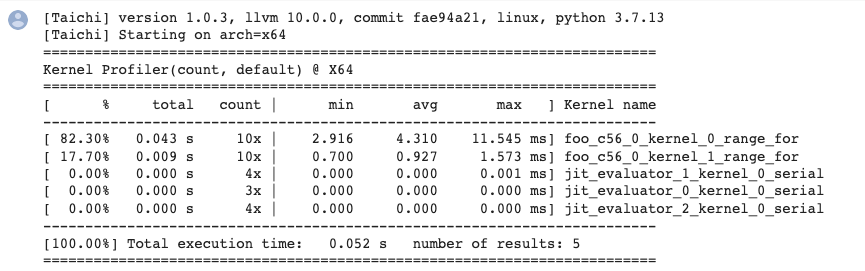To sum up:

1. A kernel that has been fully optimized by the compiler would not generate profiling records (the `bar` kernel mentioned above is a fully optimized one).

2. One kernel may generate multiple records of parallel for loops because they are divided into different tasks and assigned to separate devices.

3. Make sure you call `ti.sync()` before performance profiling if the program is running on GPU.

4. `jit_evaluator_xxx` can be ignored because it is automatically generated by the system.

5. Currently, `kernel_profiler` only supports CPU and CUDA. (But you are very encouraged to make contributions and add more backends!)

6. You are recommended to run performance profiling several times to observe the minimum or average execution time.

## Recent feature: ti.dataclass

This new feature is contributed by bsavery. It resembles `dataclasses.dataclass` introduced in Python 3.10 but functions in Taichi kernels.

A simple example of how to use this feature:

``import taichi as tiimport taichi.math as tmti.init()n_particles = 16@ti.dataclassclass Particle:    x: ti.types.vector(2, ti.f32) # position    v: ti.types.vector(2, ti.f32) # velocity        @ti.func    def at(self, t):        return self.x + self.v * t        @ti.func    def advance(self, dt):        self.x = self.at(dt)particles = Particle.field(shape=(n_particles, ))dt = 0.1@ti.kerneldef simulate():    for i in particles:        particles[i].x = tm.vec2(i, i)        particles[i].v = tm.vec2(0, 100)    for i in range(n_particles):        particles[i].advance(5)simulate()for i in range(n_particles):    print(particles[i].x)``

Result: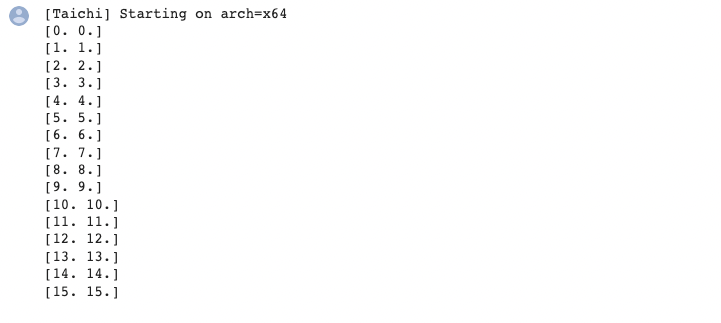Hope you have fun with the following mpm99 demo written with this new feature!

``# MPM99 using ti.dataclassimport taichi as titi.init(arch=ti.gpu)  # Try to run on GPUquality = 1  # Use a larger value for higher-res simulationsn_particles, n_grid = 9000 * quality**2, 128 * qualitydx, inv_dx = 1 / n_grid, float(n_grid)dt = 1e-4 / qualityp_vol, p_rho = (dx * 0.5)**2, 1p_mass = p_vol * p_rhoE, nu = 0.1e4, 0.2  # Young's modulus and Poisson's ratiomu_0, lambda_0 = E / (2 * (1 + nu)), E * nu / (    (1 + nu) * (1 - 2 * nu))  # Lame parameters@ti.dataclassclass Particle:    x: ti.types.vector(2, ti.f32) # position    v: ti.types.vector(2, ti.f32) # velocity    C: ti.types.matrix(2, 2, ti.f32) # affine velocity field    F: ti.types.matrix(2, 2, ti.f32) # deformation gradient    Jp: ti.f32  # plastic deformation    material: ti.i32  # material id@ti.dataclassclass Grid:    v: ti.types.vector(2, ti.f32) # grid node momentum/velocity    m: ti.f32 # velocityparticles = Particle.field(shape=(n_particles, ))grid = Grid.field(shape=(n_grid, n_grid))@ti.kerneldef substep():    for i, j in grid:        grid.v[i, j] = [0, 0]        grid.m[i, j] = 0    for p in particles:  # Particle state update and scatter to grid (P2G)        base = (particles.x[p] * inv_dx - 0.5).cast(int)        fx = particles.x[p] * inv_dx - base.cast(float)        # Quadratic kernels  [http://mpm.graphics   Eqn. 123, with x=fx, fx-1,fx-2]        w = [0.5 * (1.5 - fx)**2, 0.75 - (fx - 1)**2, 0.5 * (fx - 0.5)**2]        particles[p].F = (ti.Matrix.identity(float, 2) +                dt * particles[p].C) @ particles[p].F  # deformation gradient update        h = ti.exp(            10 *            (1.0 -             particles[p].Jp))  # Hardening coefficient: snow gets harder when compressed        if particles[p].material == 1:  # jelly, make it softer            h = 0.3        mu, la = mu_0 * h, lambda_0 * h        if particles[p].material == 0:  # liquid            mu = 0.0        U, sig, V = ti.svd(particles[p].F)        J = 1.0        for d in ti.static(range(2)):            new_sig = sig[d, d]            if particles[p].material == 2:  # Snow                new_sig = ti.min(ti.max(sig[d, d], 1 - 2.5e-2),                                 1 + 4.5e-3)  # Plasticity            particles[p].Jp *= sig[d, d] / new_sig            sig[d, d] = new_sig            J *= new_sig        if particles[p].material == 0:  # Reset deformation gradient to avoid numerical instability            particles.F[p] = ti.Matrix.identity(float, 2) * ti.sqrt(J)        elif particles[p].material == 2:            particles.F[p] = U @ sig @ V.transpose(            )  # Reconstruct elastic deformation gradient after plasticity        stress = 2 * mu * (particles[p].F - U @ V.transpose()) @ particles[p].F.transpose(        ) + ti.Matrix.identity(float, 2) * la * J * (J - 1)        stress = (-dt * p_vol * 4 * inv_dx * inv_dx) * stress        affine = stress + p_mass * particles.C[p]        for i, j in ti.static(ti.ndrange(                3, 3)):  # Loop over 3x3 grid node neighborhood            offset = ti.Vector([i, j])            dpos = (offset.cast(float) - fx) * dx            weight = w[i] * w[j]            grid.v[base + offset] += weight * (p_mass * particles.v[p] + affine @ dpos)            grid.m[base + offset] += weight * p_mass    for i, j in grid:        if grid.m[i, j] > 0:  # No need for epsilon here            grid.v[i,                   j] = (1 / grid.m[i, j]) * grid.v[i,                                                    j]  # Momentum to velocity            grid.v[i, j] -= dt * 50  # gravity            if i < 3 and grid.v[i, j] < 0:                grid.v[i, j] = 0  # Boundary conditions            if i > n_grid - 3 and grid.v[i, j] > 0: grid.v[i, j] = 0            if j < 3 and grid.v[i, j] < 0: grid.v[i, j] = 0            if j > n_grid - 3 and grid.v[i, j] > 0: grid.v[i, j] = 0    for p in particles.x:  # grid to particle (G2P)        base = (particles.x[p] * inv_dx - 0.5).cast(int)        fx = particles.x[p] * inv_dx - base.cast(float)        w = [0.5 * (1.5 - fx)**2, 0.75 - (fx - 1.0)**2, 0.5 * (fx - 0.5)**2]        new_v = ti.Vector.zero(float, 2)        new_C = ti.Matrix.zero(float, 2, 2)        for i, j in ti.static(ti.ndrange(                3, 3)):  # loop over 3x3 grid node neighborhood            dpos = ti.Vector([i, j]).cast(float) - fx            g_v = grid.v[base + ti.Vector([i, j])]            weight = w[i] * w[j]            new_v += weight * g_v            new_C += 4 * inv_dx * weight * g_v.outer_product(dpos)        particles.v[p], particles.C[p] = new_v, new_C        particles.x[p] += dt * particles.v[p]  # advectiongroup_size = n_particles // 3@ti.kerneldef initialize():    for i in range(n_particles):        particles[i].x = [            ti.random() * 0.2 + 0.3 + 0.10 * (i // group_size),            ti.random() * 0.2 + 0.05 + 0.32 * (i // group_size)        ]        particles[i].material = i // group_size  # 0: fluid 1: jelly 2: snow        particles[i].v = ti.Matrix([0, 0])        particles[i].F = ti.Matrix([[1, 0], [0, 1]])        particles[i].Jp = 1def main():    initialize()    gui = ti.GUI("Taichi MLS-MPM-99", res=512, background_color=0x112F41)    for i in range(400):        for s in range(int(2e-3 // dt)):            substep()        gui.circles(particles.x.to_numpy(),                    radius=1.5,                    palette=[0x068587, 0xED553B, 0xEEEEF0],                    palette_indices=particles.material)        gui.show()if __name__ == '__main__':    main()``

You are most welcome to give us your opinions on how to improve Taichi's features by opening an issue on GitHub: https://github.com/taichi-dev/taichi